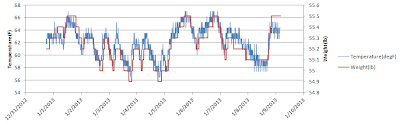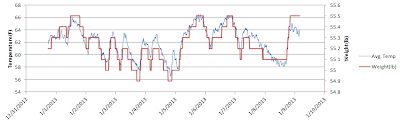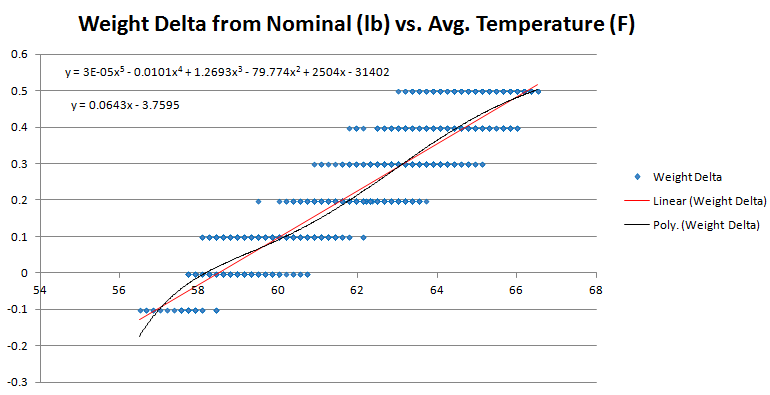## Tuesday, January 22, 2013

### First scale test results

I took some time to analyze the results from my scale test.  If you like graphs, then keep reading.  If you don't like graphs then skip to my conclusion at the bottom.  I happen to like graphs...

To summarize the previous post, I wanted to see if there were two effects happening to my fancy new RS232 scale: 1) temperature and 2) sensor drift (or creep).  The easiest way to track this was to measure the weight of an object with a constant mass over time, while also measuring temperature over the same time span.  I did that and now have a week's worth of measurement data to sift through.Figure 1: Temperature and Weight vs. Time
First I graphed weight and temperature each versus time.  Figure 1 shows this graph.  You can immediately see that the measured weight correlates closely with the temperature, so clearly I was right that there is a temperature effect.  The second thing to note is that the weight line shows clear quantization, but this is to be expected as the scale only specifies to have a 0.1 lb resolution (but it's still nice to see it clearly in the graph).  Another thing to note is that the temperature is pretty noisy.Figure 2: Average Temperature and Weight vs. Time
Figure 2 is the same graph but with a moving averaged temperature.  Perhaps a "moving average" is not the correct term for this; I never did much with formal statistics.  I basically just calculated each reading by taking the mean of the previous 6 readings.  You can see the temperature line looks much less noisy now and the correlation between temperature and weight still remains.Figure 3
My next task was to try and figure out the mathematical relationship between weight and temperature, so that I can then (hopefully) correct for temperature and get a more accurate weight reading.  The graph for weight versus temperature is shown in Figure 3.  There are several interesting things here.  The first is that you can see that both the weight and the temperature are quantized since all the blue points in the graph come out on grid lines.  The next thing to note is that the point cloud is pretty widely distributed (which isn't good).  I had Excel generate a best fit linear trend line.Figure 4
Next up I repeated that graph (Figure 4) but with the the averaged temperature data from Figure 2.  Now you can see that the quantization in the temperature data has disappeared but remains for the weight, which is what we'd expect.  The trend line has changed a little based on this adjustment.  I also threw a 6th order polynomial fit trend line on the data to see if that would fit the data better, but as you can see that is nearly a straight line, so we'll stick with the linear fit line for future calculations since it seems to be a pretty good approximation (at least compared with a polynomial fit).Figure 5
Now from this we can use the calculated trend line to create a mathematical attempt at canceling out the temperature effect of the scale readings.  Figure 5 is a graph of the temperature-adjusted weight plotted over time.  The ideal line would be a line that is constant at 55 lb.  The adjusted weight line is clearly not a straight line but if you compare it to Figure 2 we have certainly made an improvement over the raw weight readings.

As it stands this temperature correction is probably useful enough that I could start using the scale for continuous weight measurements, however I'd like to make it better if I can.  I think one improvement I can make (which I mentioned in my previous post) was that my temperature sensor has pretty low resolution (about 1 degree Fahrenheit). I suspect that having a sensor with 0.1 degree resolution would help, and might bring the point cloud in Figure 3 into a tighter cloud which would make the generated trend line more accurate.  So now I'm going to start looking around for a more suitable temperature sensor (I might already have one on hand in my parts library) and re-run the test to gather fresh data.